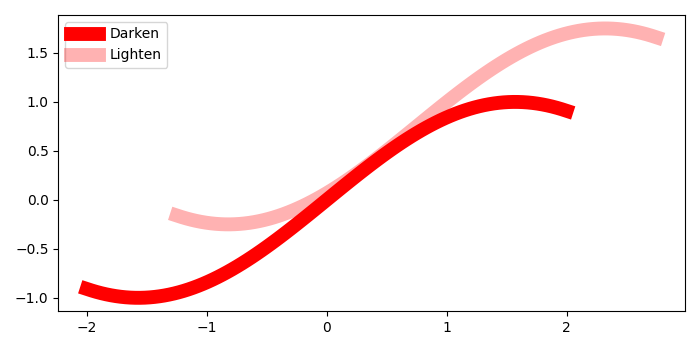# Darken or lighten a color in Matplotlib

To darken and lighten the color, we can chage the alpha value in the argument of plot() method.Greater the aplha value, darker will be the color.

## Steps

• Create data points for xs and ys using numpy.
• Plot two lines with different value of alpha, to replicate darker and lighter color of the lines
• Place legend of the plot using legend() method.
• To display the figure, use show() method.

## Example

import numpy as np
from matplotlib import pyplot as plt
plt.rcParams["figure.figsize"] = [7.00, 3.50]
plt.rcParams["figure.autolayout"] = True
xs = np.linspace(-2, 2, 100)
ys = np.sin(xs)
plt.plot(xs, ys, c='red', lw=10, label="Darken")
plt.plot(xs+.75, ys+.75, c='red', lw=10, alpha=0.3, label="Lighten")
plt.legend(loc='upper left')
plt.show()

## Output